## 4.2 Thermal structure

Table 7 lists the mean electron temperatures of the entire nebula in the models MC1 and MC2 weighted by ionic species, from the neutral (I) to the highly ionized ions (VII). The definition for the weighted-mean temperatures was given in Ercolano et al. (2003c). The value ofNII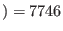K predicted by the model MC1 is about 1150K lower than the value ofNII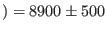K empirically derived from CELs by García-Rojas et al. (2009). This could be due to recombination contributions to the auroral line. The recombination contribution estimated (using Eq. 1 in Liu et al., 2000) about 12 percent in the model MC1 is much lower to explain the measured intensity of the [NII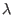5755 line. However, the model MC2 predicts the [NII5755 line to be 40 percent higher than the value derived from the model MC1 (see recombination contribution estimated by Eq. 1 in Table 6). We also notice that the [NII] temperature is roughly equal to the [OIII] temperature in low excitation PNe (Kingsburgh & Barlow, 1994), so the empirical value of the [NII] electron temperature derived by García-Rojas et al. (2009) is difficult to be explained. The temperature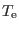(OIIK predicted by the model MC1 is about 670k higher thanOIIK empirically derived by García-Rojas et al. (2009), while(OII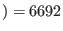K predicted by the model MC2 is about 360k lower than the empirical value. Moreover, the temperature of [OIII] calculated from the model MC1,OIIIK, is about 710K higher the empirical result ofOIIIK, whereasOIIIK predicted by the model MC2 is about 330K lower the empirical value.

Table 8 presents the electron temperatures of the different components of the model MC3 weighted by ionic abundances, as well as the mean temperatures of the entire nebula. The first entries for each element are for the normal abundance plasma, the second entries are for the metal-rich inclusion, and the third entries are for the entire nebula (including both the normal and the H-poor components). It can be seen that the temperatures weighted by ionic abundances in the two different components of the nebula are very different. The electron temperatures separately weighted by the ionic species of the metal-rich inclusions were much lower than those from the normal part. The temperature ofNIIK predicted by the normal component of the model MC3 is about 1590K lower than the value empirically derived. However,(OII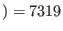K and(OIIIK obtained by the normal component of the model MC3 are in reasonable agreement with the values empirically derived by García-Rojas et al. (2009). We see thatHeI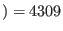K weighted by the metal-rich component of the model MC3 is lower than the empirical value,HeI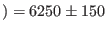K (García-Rojas et al., 2009), whereasHeI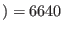K weighted by the whole nebula is about 390K higher the empirical value Moreover,HIK weighted by the metal-rich component andHIK weighted by the whole nebula are reasonably in the range ofHI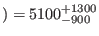K empirically derived from the Balmer Jump to H11 flux ratio (García-Rojas et al., 2009). We take no account for the interaction between the two components, namely normal and metal-rich, which could also lead to a temperature variation. Note that the radiative transfer in a neutral region is not currently supported by MOCASSIN, so the code only estimates temperatures that likely correspond to a potential narrow transition region between ionized and neutral regions for a radiation-bounded object. Neutral elements in this PN are negligible (see Table 9), so the temperatures of the neutral species listed in Table 8 do not have any significant physical meaning. We also see that the two components have a local thermal pressure ratio of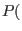metal-rich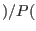normal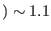, which means each metal-rich cell is in pressure equilibrium with its surrounding normal gas. The higher thermal pressure forces the dense, metal-rich knots to expand and reduce their density and temperature during the evolution phase of the nebula.

Ashkbiz Danehkar
2018-03-28